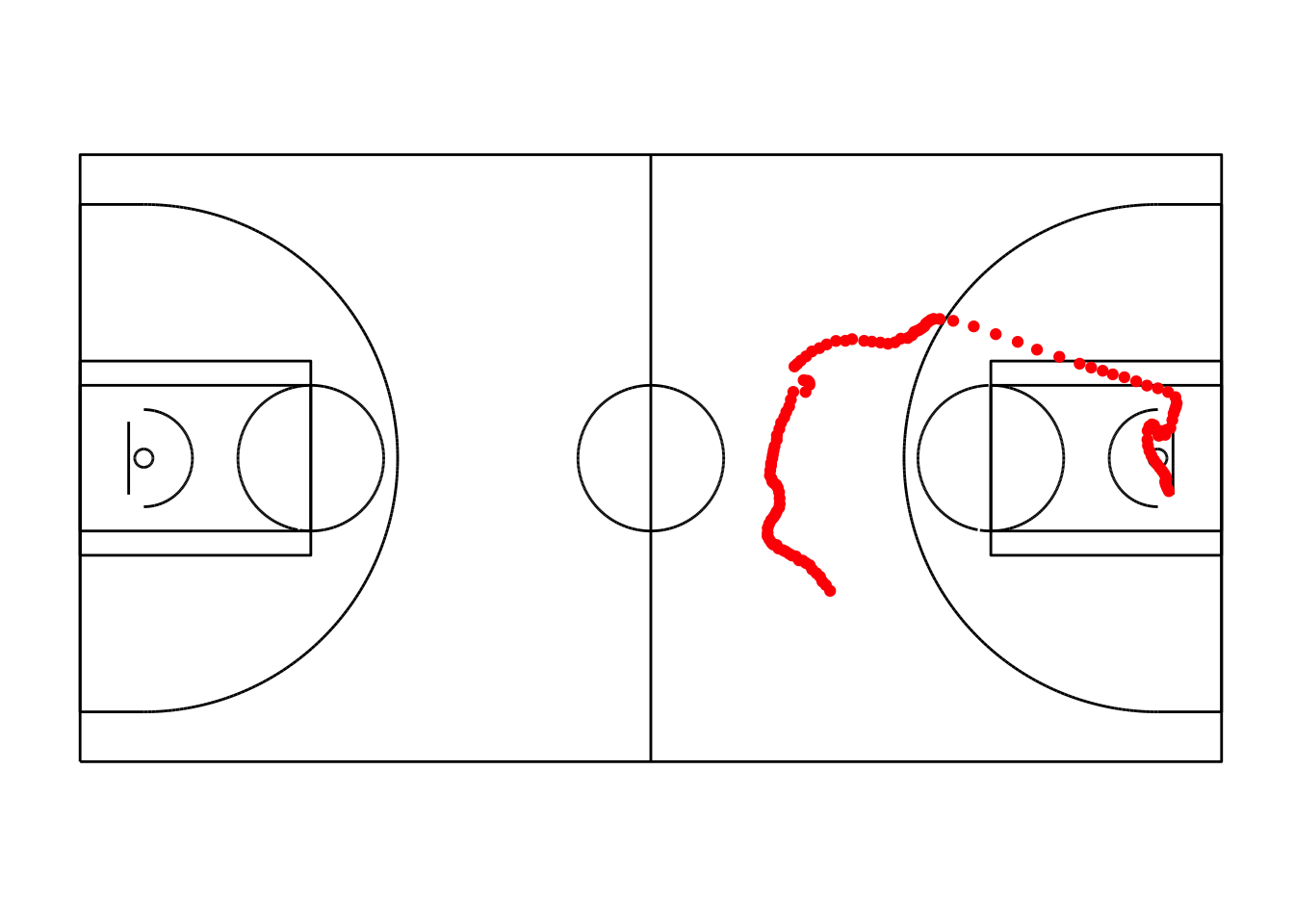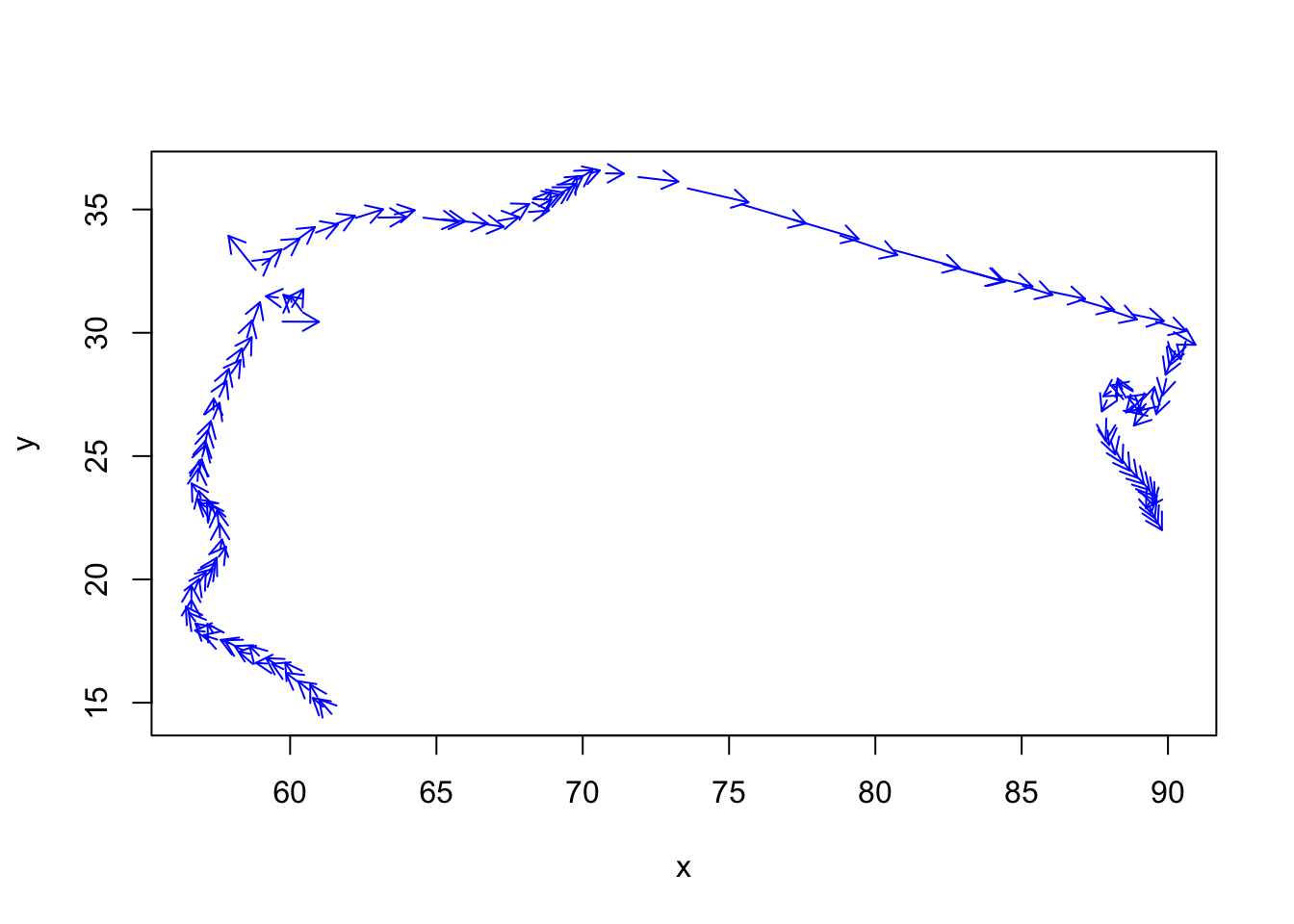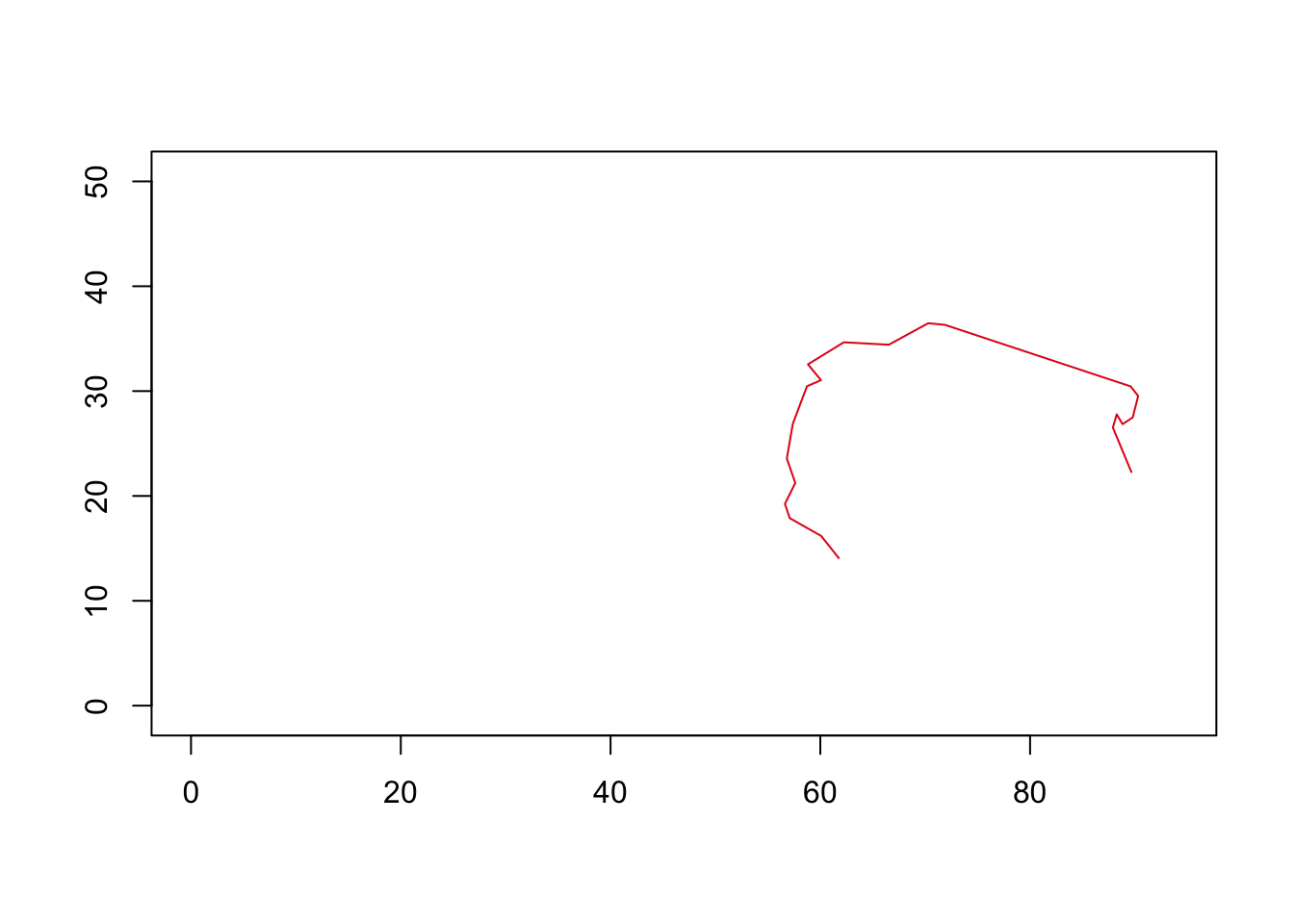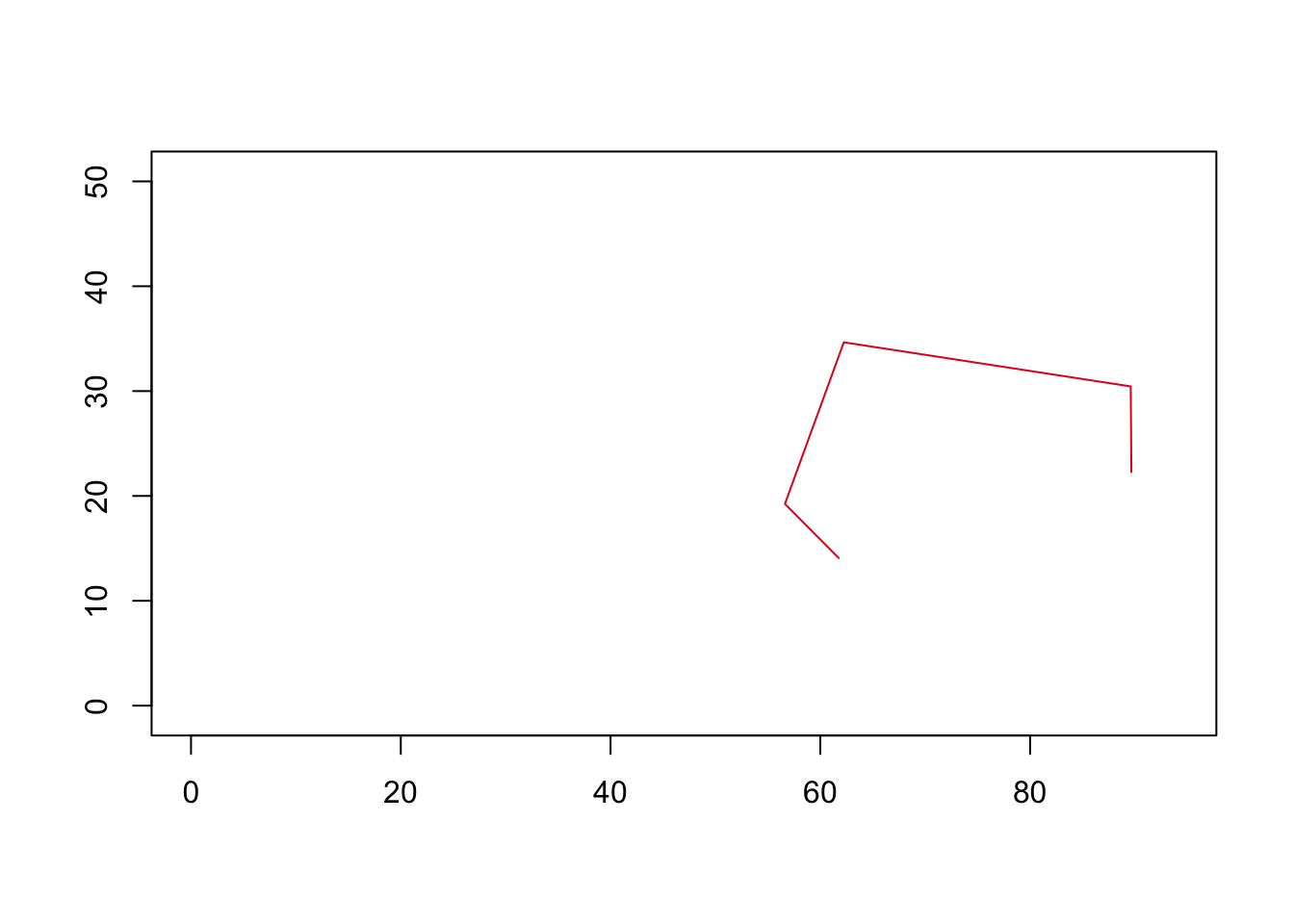This page shares a few different ways to analyzing player and ball trajectories. I have been exploring these methods as I build new features on the SportVu data. I start by visualizing trajectories, then simplifying trajectories, and finally consider some similarity measures.

The inspiration for this work was the hierarchical clustering of plays by Johannes Becker in this article at Nylon Calculus.

As a starting point, it is necessary to use my previous notebooks to grab the data.

``````library(dplyr)
library(fields)
library(spacetime)
library(rgeos)
library(sp)
library(SimilarityMeasures)
source("_functions.R")
source("_function_fullcourt.R")``````

### Grab the data for one event

Lets start by using the quick pass in the Magic Wizards game on January 1st (event ID of 422). You can see the youtube video or the SportVU movement data not currently available.

The first step is extracting the data for event ID 422. Please refer to my other posts for how this data is downloaded and merged. I am importing a file that has been previously processed in a data frame with movement data.

``````all.movements <- read.csv("data/0021500490.gz")
event_df <- all.movements %>%
dplyr::arrange(quarter,desc(game_clock),x_loc) %>%
filter(event.id==422)``````

### Viewing the motion of the ball

``````df_ball <- event_df %>%
filter(player_id == "-1") %>%
filter (shot_clock != 24)  #Remove some extra spillover data from the next event
#Plot ball movement
fullcourt() +
geom_point(data=df_ball,aes(x=x_loc,y=y_loc),color='red') ``````## Vector Fields

The SportVu data provides a trajectory of movement. Vector fields can be used to analyze and visualize the direction and speed of the ball. The arrow plot uses the location and velocity for the plot.

``````# Using fields package
x <- df_ball\$x_loc
y <- df_ball\$y_loc
u <- diff(x)
v <- diff(y)
x <- x[-1]
y <- y[-1]
plot( x,y, type="n")
arrow.plot(x,y,u,v, arrow.ex=.1, length=.1, col='blue', lwd=1)``````### Simplifying Trajectories

The SportVu data has a frequency of 25 times a second. While this granularity provides lots of detail, sometimes there is a need to simplify. The Ramer–Douglas–Peucker algorithm (RDP) is a well known algorithm for reducing the number of points in a curve. Using this with the fields package, means first we need to convert the data into a SpatialLines object.

``````# Get data into spatial lines
xy <- cbind(df_ball\$x_loc, df_ball\$y_loc)
xy.sp <- SpatialPoints(xy)
sl = as(xy.sp, "SpatialLines")
# Applies RDP
xy.spdf.simple <- gSimplify(sl,tol = .5,topologyPreserve=FALSE)
#See how much the data has been simplified
plot(xy.spdf.simple, pch = 2,xlim = c(0,94),ylim=c(0,50),axes = TRUE)````````````##By changing the tolerance value, we can affect how much it is simplified
xy.spdf.simple <- gSimplify(sl,tol = 5,topologyPreserve=FALSE)
plot(xy.spdf.simple, pch = 2,xlim = c(0,94),ylim=c(0,50),axes = TRUE)``````### Analyze two trajectories

For this example, I am taking one event and splitting into two trajectories, to illustrate this approach.

``````# Get the trajectories to use
event_df2 <- all.movements %>%
dplyr::arrange(quarter,desc(game_clock),x_loc) %>%
filter(player_id == "-1") %>%
filter(event.id==35)
df1 <- event_df2 %>% filter(game_clock<=397.84)
df2 <- event_df2 %>% filter(game_clock>397.84)

# Plot first trajectory
fullcourt() + geom_point(data=df1,aes(x=x_loc,y=y_loc),color='red') ``````